# IFC 4.3.x development

## 8.20.3.4 IfcEdge

### 8.20.3.4.1 Semantic definitions at the entity

An IfcEdge defines two vertices being connected topologically. The geometric representation of the connection between the two vertices defaults to a straight line if no curve geometry is assigned using the subtype IfcEdgeCurve. The IfcEdge can therefore be used to exchange straight edges without an associated geometry provided by IfcLine or IfcPolyline thought IfcEdgeCurve.EdgeGeometry.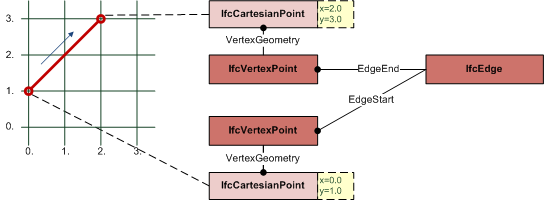EXAMPLE  Figure 1 illustrates an example where the bounds of the IfcEdge are given by the EdgeStart and EdgeEnd; this also determines the direction of the edge. The location within a coordinate space is determined by the IfcVertexPoint type for EdgeStart and EdgeEnd. Since no edge geometry is assigned, it defaults to a straight line agreeing to the direction sense. Figure 1 — Edge representation

NOTE  Definition according to ISO/CD 10303-42:1992
An edge is the topological construct corresponding to the connection of two vertices. More abstractly, it may stand for a logical relationship between two vertices. The domain of an edge, if present, is a finite, non-self-intersecting open curve in R^M^, that is, a connected 1-dimensional manifold. The bounds of an edge are two vertices, which need not be distinct. The edge is oriented by choosing its traversal direction to run from the first to the second vertex. If the two vertices are the same, the edge is a self loop. The domain of the edge does not include its bounds, and 0 ≤ Ξ ≤ ∞. Associated with an edge may be a geometric curve to locate the edge in a coordinate space; this is represented by the edge curve subtype. The curve shall be finite and non-self-intersecting within the domain of the edge. An edge is a graph, so its multiplicity M and graph genus G^e^ may be determined by the graph traversal algorithm. Since M = E = 1, the Euler equation (1) reduces in the case to: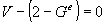where V = 1 or 2, and G^e^ = 1 or 0. Specifically, the topological edge defining data shall satisfy: > * an edge has two vertices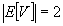* the vertices need not be distinct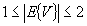* Equation shall hold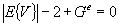NOTE  Entity adapted from edge defined in ISO 10303-42.

HISTORY  New entity in IFC2.0

Informal Propositions:

1. The edge has dimensionality 1.
2. The extent of an edge shall be finite and nonzero.

### 8.20.3.4.1 Attributes

# Attribute Type Description
IfcRepresentationItem
LayerAssignmentSET [0:1] OF IfcPresentationLayerAssignment FOR AssignedItemsAssignment of the representation item to a single or multiple layer(s). The LayerAssignments can override a LayerAssignments of the IfcRepresentation it is used within the list of Items.

> IFC2x3 CHANGE  The inverse attribute LayerAssignments has been added.

> IFC4 CHANGE  The inverse attribute LayerAssignment has been restricted to max 1. Upward compatibility for file based exchange is guaranteed.
StyledByItem SET [0:1] OF IfcStyledItem FOR Item Reference to the IfcStyledItem that provides presentation information to the representation, e.g. a curve style, including colour and thickness to a geometric curve.

> IFC2x3 CHANGE  The inverse attribute StyledByItem has been added.
IfcTopologicalRepresentationItem
IfcEdge
1 EdgeStart IfcVertex Start point (vertex) of the edge.
2 EdgeEnd IfcVertex End point (vertex) of the edge. The same vertex can be used for both EdgeStart and EdgeEnd.

### 8.20.3.4.3 Formal representations

```ENTITY IfcEdge
SUPERTYPE OF (ONEOF
(IfcEdgeCurve
,IfcOrientedEdge
,IfcSubedge))
SUBTYPE OF (IfcTopologicalRepresentationItem);
EdgeStart : IfcVertex;
EdgeEnd : IfcVertex;
END_ENTITY;```